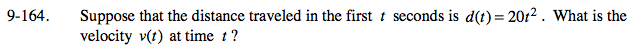### Home > PC > Chapter 9 > Lesson 9.3.5 > Problem9-164

9-164.

Suppose that the distance traveled in the first t seconds is d(t) = 20t2. What is the velocity v(t) at time t? Homework Help ✎What is the slope function for d(t)?

v(t) = 40t